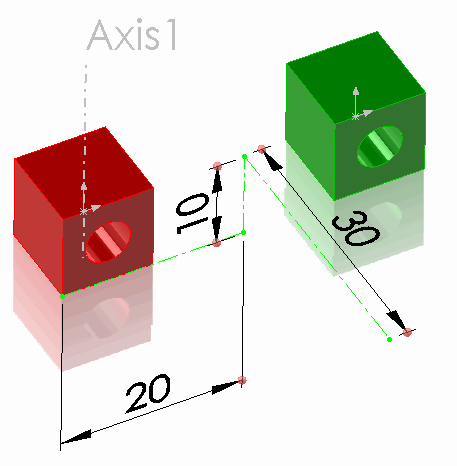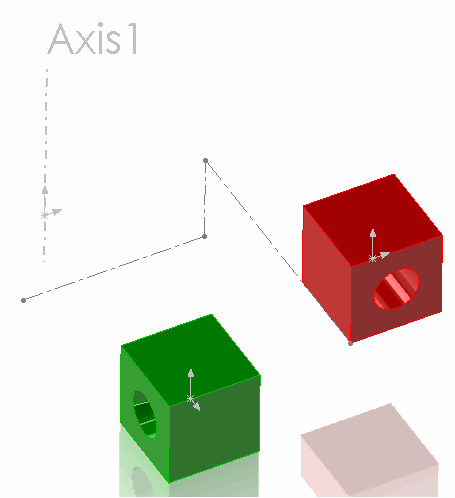# Load components presentation transforms from CSV file using SOLIDWORKS APIComponents in the original positions

This example demonstrates how to load the transformation matrix for the components from the CSV file and apply it as a presentation transform using SOLIDWORKS API

• Download and open the Example SOLIDWORKS Files
• Download CSV File and save to disc
• Modify the path to the CSV file in the macro constant
• Run the macro. Macro stops the execution and the components are transformed as shown belowComponents in the transformed position

Red component is translated in XYZ space and green component is rotated 90 degrees around global Y axis (Axis 1).

Please note that the components are moved regardless the fact that both of them are fully defined in space (by mates or fix constraint). And the mates are still preserved. The reason is that the presentation transform was applied instead of the permanent one. This allows to only move the components for visual purposes without changing the geometry.

Continue the macro with F5 or Play button to remove the presentation transformation. Use IComponent2::Transform2 SOLIDWORKS API property instead of IComponent2::PresentationTransform to apply the permanent transform if needed (in this case it is required to remove any mates which will not fit this transformation).

```Const INPUT_FILE_PATH = "D:\transforms.csv"

Dim swApp As SldWorks.SldWorks
Dim swAssy As SldWorks.AssemblyDoc

Sub main()

On Error GoTo Error

Set swApp = Application.SldWorks

Set swAssy = swApp.ActiveDoc

Dim vTable As Variant
vTable = ReadCsvFile(INPUT_FILE_PATH, True)

swAssy.EnablePresentation = True
PreviewComponentsPosition vTable

Error:
Stop
swAssy.EnablePresentation = False

End Sub

Function ReadCsvFile(filePath As String, firstRowHeader As Boolean) As Variant

'rows x columns
Dim vTable() As Variant

On Error GoTo Error

Dim fileName As String
Dim tableRow As String
Dim fileNo As Integer

fileNo = FreeFile

Open filePath For Input As #fileNo

Dim isFirstRow As Boolean
Dim isTableInit As Boolean

isFirstRow = True
isTableInit = False

Do While Not EOF(fileNo)

Line Input #fileNo, tableRow

If Not isFirstRow Or Not firstRowHeader Then

Dim vCells As Variant
vCells = Split(tableRow, ",")

Dim lastRowIndex As Integer

If Not isTableInit Then
lastRowIndex = 0
isTableInit = True
ReDim Preserve vTable(lastRowIndex)
Else
lastRowIndex = UBound(vTable, 1) + 1
ReDim Preserve vTable(lastRowIndex)
End If

vTable(lastRowIndex) = vCells

End If

If isFirstRow Then
isFirstRow = False
End If

Loop

Close #fileNo

ReadCsvFile = vTable

Exit Function

Error:

ReadCsvFile = Empty

End Function

Sub PreviewComponentsPosition(table As Variant)

Dim i As Integer

For i = 0 To UBound(table)

Dim swComp As SldWorks.Component2

Dim compName As String
compName = table(i)(0)

Set swComp = GetComponent(compName)

If Not swComp Is Nothing Then
swComp.RemovePresentationTransform
swComp.PresentationTransform = CreateTransform(table(i))
Else
Debug.Print compName & " is not found"
End If

Next

Dim swModelView As SldWorks.ModelView
Set swModelView = swAssy.ActiveView
swModelView.GraphicsRedraw Nothing

End Sub

Function CreateTransform(tableRow As Variant) As SldWorks.MathTransform

Dim swMathUtils As SldWorks.MathUtility
Set swMathUtils = swApp.GetMathUtility

Dim dMatrix(15) As Double

dMatrix(0) = CDbl(tableRow(1)): dMatrix(1) = CDbl(tableRow(2)): dMatrix(2) = CDbl(tableRow(3)): dMatrix(3) = CDbl(tableRow(5))
dMatrix(4) = CDbl(tableRow(6)): dMatrix(5) = CDbl(tableRow(7)): dMatrix(6) = CDbl(tableRow(9)): dMatrix(7) = CDbl(tableRow(10))
dMatrix(8) = CDbl(tableRow(11)): dMatrix(9) = CDbl(tableRow(13)): dMatrix(10) = CDbl(tableRow(14)): dMatrix(11) = CDbl(tableRow(15))
dMatrix(12) = CDbl(tableRow(16)): dMatrix(13) = CDbl(tableRow(4)): dMatrix(14) = CDbl(tableRow(8)): dMatrix(15) = CDbl(tableRow(12))

Dim swXform As SldWorks.MathTransform
Set swXform = swMathUtils.CreateTransform(dMatrix)

Set CreateTransform = swXform

End Function

Function GetComponent(compPath As String) As Component2

Dim swComp As SldWorks.Component2

Dim compNames As Variant
compNames = Split(compPath, "\")

Dim i As Integer
Set swComp = swAssy.ConfigurationManager.ActiveConfiguration.GetRootComponent()

For i = 0 To UBound(compNames)
If Not swComp Is Nothing Then

Dim vChildComps As Variant
Dim j As Integer

vChildComps = swComp.GetChildren

Dim isCompFound As Boolean
isCompFound = False

If Not IsEmpty(vChildComps) Then

Dim shortCompName As String

For j = 0 To UBound(vChildComps)

Dim swChildComp As SldWorks.Component2
Set swChildComp = vChildComps(j)

Dim vShortNames As Variant
vShortNames = Split(swChildComp.Name2, "/")
shortCompName = vShortNames(UBound(vShortNames))

If LCase(shortCompName) = LCase(compNames(i)) Then
Set swComp = swChildComp
isCompFound = True
End If
Next
End If

If Not isCompFound Then
Set swComp = Nothing
End If

End If
Next

Set GetComponent = swComp

End Function

```